CHAPTER 1 Whole Numbers, Integers, and Introduction to Algebra

# 1.1 Whole Numbers

Learning Objectives

By the end of this section, you will be able to:

• Use place value with whole numbers
• Identify multiples and and apply divisibility tests
• Find prime factorization and least common multiples

As we begin our study of intermediate algebra, we need to refresh some of our skills and vocabulary. This chapter and the next will focus on whole numbers, integers, fractions, decimals, and real numbers. We will also begin our use of algebraic notation and vocabulary.

# Use Place Value with Whole Numbers

The most basic numbers used in algebra are the numbers we use to count objects in our world: 1, 2, 3, 4, and so on. These are called the counting numbers. Counting numbers are also called natural numbers. If we add zero to the counting numbers, we get the set of whole numbers.Counting Numbers: 1, 2, 3, …Whole Numbers: 0, 1, 2, 3, …

The notation “…” is called ellipsis and means “and so on,” or that the pattern continues endlessly.

We can visualize counting numbers and whole numbers on a number line .See Figure 1.

The numbers on the number line get larger as they go from left to right, and smaller as they go from right to left. While this number line shows only the whole numbers 0 through 6, the numbers keep going without end.

Our number system is called a place value system, because the value of a digit depends on its position in a number. Figure 2 shows the place values. The place values are separated into groups of three, which are called periods. The periods are ones, thousands, millions, billions, trillions, and so on. In a written number, commas separate the periods.

The number 5,278,194 is shown in the chart. The digit 5 is in the millions place. The digit 2 is in the hundred-thousands place. The digit 7 is in the ten-thousands place. The digit 8 is in the thousands place. The digit 1 is in the hundreds place. The digit 9 is in the tens place. The digit 4 is in the ones place.

EXAMPLE 1

In the number 63,407,218, find the place value of each digit:

1. 7
2. 0
3. 1
4. 6
5. 3
Solution

Place the number in the place value chart:a) The 7 is in the thousands place.
b) The 0 is in the ten thousands place.
c) The 1 is in the tens place.
d) The 6 is in the ten-millions place.
e) The 3 is in the millions place.

TRY IT 1.1

For the number 27,493,615, find the place value of each digit:

a) 2 b) 1 c) 4 d) 7 e) 5

a) ten millions b) tens c) hundred thousands d) millions e) ones

TRY IT 1.2

For the number 519,711,641,328, find the place value of each digit:

a) 9 b) 4 c) 2 d) 6 e) 7

a) billions b) ten thousands c) tens d) hundred thousands e) hundred millions

When you write a check, you write out the number in words as well as in digits. To write a number in words, write the number in each period, followed by the name of the period, without the s at the end. Start at the left, where the periods have the largest value. The ones period is not named. The commas separate the periods, so wherever there is a comma in the number, put a comma between the words (see Figure 3). The number 74,218,369 is written as seventy-four million, two hundred eighteen thousand, three hundred sixty-nine.

HOW TO: Name a Whole Number in Words.

1. Start at the left and name the number in each period, followed by the period name.
2. Put commas in the number to separate the periods.
3. Do not name the ones period.

EXAMPLE 2

Name the number 8,165,432,098,710 using words.

Solution

Name the number in each period, followed by the period name.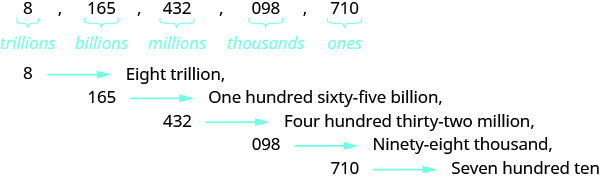Put the commas in to separate the periods.

So,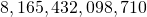is named as eight trillion, one hundred sixty-five billion, four hundred thirty-two million, ninety-eight thousand, seven hundred ten.

TRY IT 2.1

Name the number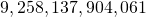using words.

nine trillion, two hundred fifty-eight billion, one hundred thirty-seven million, nine hundred four thousand, sixty-one

TRY IT 2.2

Name the number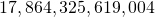using words.

seventeen trillion, eight hundred sixty-four billion, three hundred twenty-five million, six hundred nineteen thousand four

We are now going to reverse the process by writing the digits from the name of the number. To write the number in digits, we first look for the clue words that indicate the periods. It is helpful to draw three blanks for the needed periods and then fill in the blanks with the numbers, separating the periods with commas.

HOW TO: Write a Whole Number Using Digits.

1. Identify the words that indicate periods. (Remember, the ones period is never named.)
2. Draw three blanks to indicate the number of places needed in each period. Separate the periods by commas.
3. Name the number in each period and place the digits in the correct place value position.

EXAMPLE 3

Write nine billion, two hundred forty-six million, seventy-three thousand, one hundred eighty-nine as a whole number using digits.

Solution

Identify the words that indicate periods.
Except for the first period, all other periods must have three places. Draw three blanks to indicate the number of places needed in each period. Separate the periods by commas.
Then write the digits in each period.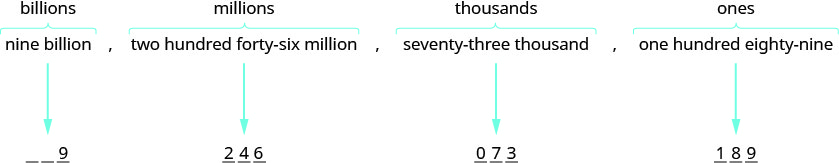The number is 9,246,073,189.

TRY IT 3.1

Write the number two billion, four hundred sixty-six million, seven hundred fourteen thousand, fifty-one as a whole number using digits.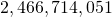TRY IT 3.2

Write the number eleven billion, nine hundred twenty-one million, eight hundred thirty thousand, one hundred six as a whole number using digits.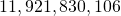In 2016, Statistics Canada estimated the population of Toronto as 13,448,494. We could say the population of Toronto was approximately 13.4 million. In many cases, you don’t need the exact value; an approximate number is good enough.

The process of approximating a number is called rounding. Numbers are rounded to a specific place value, depending on how much accuracy is needed. Saying that the population of Toronto is approximately 13.4 million means that we rounded to the hundred thousands place.

EXAMPLE 4

Round 23,658 to the nearest hundred.

Solution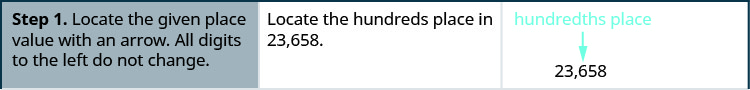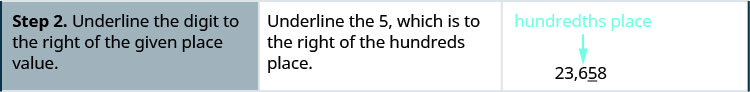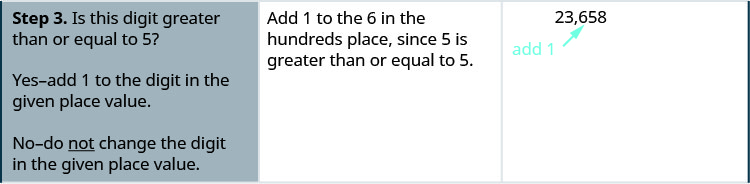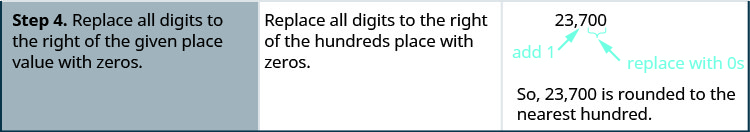TRY IT 4.1

Round to the nearest hundred: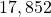.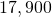TRY IT 4.2

Round to the nearest hundred: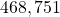.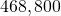HOW TO: Round Whole Numbers.

1. Locate the given place value and mark it with an arrow. All digits to the left of the arrow do not change.
2. Underline the digit to the right of the given place value.
3. Is this digit greater than or equal to 5?
• Yes–addto the digit in the given place value.
• No–do not change the digit in the given place value.
4. Replace all digits to the right of the given place value with zeros.

EXAMPLE 5

Round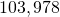to the nearest:

1. hundred
2. thousand
3. ten thousand
Solution

a)

 Locate the hundreds place in 103,978.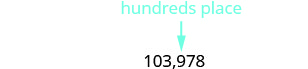Underline the digit to the right of the hundreds place.Since 7 is greater than or equal to 5, add 1 to the 9. Replace all digits to the right of the hundreds place with zeros.So, 104,000 is 103,978 rounded to the nearest hundred.

b)

 Locate the thousands place and underline the digit to the right of the thousands place.Since 9 is greater than or equal to 5, add 1 to the 3. Replace all digits to the right of the hundreds place with zeros.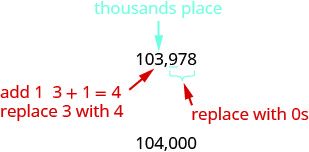So, 104,000 is 103,978 rounded to the nearest thousand.

c)

 Locate the ten thousands place and underline the digit to the right of the ten thousands place.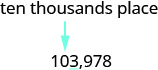Since 3 is less than 5, we leave the 0 as is, and then replace the digits to the right with zeros.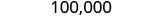So, 100,000 is 103,978 rounded to the nearest ten thousand.

TRY IT 5.1

Round 206,981 to the nearest: a) hundred b) thousand c) ten thousand.

a) 207,000 b) 207,000 c) 210,000

TRY IT 5.2

Round 784,951 to the nearest: a) hundred b) thousand c) ten thousand.

a) 785,000 b) 785,000 c) 780,000

# Identify Multiples and Apply Divisibility Tests

The numbers 2, 4, 6, 8, 10, and 12 are called multiples of 2. A multiple of 2 can be written as the product of a counting number and 2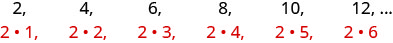Similarly, a multiple of 3 would be the product of a counting number and 3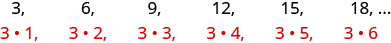We could find the multiples of any number by continuing this process.

The Table 1 below shows the multiples of 2 through 9 for the first 12 counting numbers.

Table 1
Counting Number 1 2 3 4 5 6 7 8 9 10 11 12
Multiples of 2 2 4 6 8 10 12 14 16 18 20 22 24
Multiples of 3 3 6 9 12 15 18 21 24 27 30 33 36
Multiples of 4 4 8 12 16 20 24 28 32 36 40 44 48
Multiples of 5 5 10 15 20 25 30 35 40 45 50 55 60
Multiples of 6 6 12 18 24 30 36 42 48 54 60 66 72
Multiples of 7 7 14 21 28 35 42 49 56 63 70 77 84
Multiples of 8 8 16 24 32 40 48 56 64 72 80 88 96
Multiples of 9 9 18 27 36 45 54 63 72 81 90 99 108
Multiples of 10 10 20 30 40 50 60 70 80 90 100 110 120

Multiple of a Number

A number is a multiple of n if it is the product of a counting number and n.

Another way to say that 15 is a multiple of 3 is to say that 15 is divisible by 3. That means that when we divide 3 into 15, we get a counting number. In fact,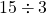is 5, so 15 is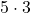.

Divisible by a Number

If a number m is a multiple of n, then m is divisible by n.

Look at the multiples of 5 in Table 1. They all end in 5 or 0. Numbers with last digit of 5 or 0 are divisible by 5. Looking for other patterns in Table 1 that shows multiples of the numbers 2 through 9, we can discover the following divisibility tests:

Divisibility Tests

A number is divisible by:

• 2 if the last digit is 0, 2, 4, 6, or 8.
• 3 if the sum of the digits is divisible by 3.
• 5 if the last digit is 5 or 0.
• 6 if it is divisible by both 2 and 3.
• 10 if it ends with 0.

EXAMPLE 6

Is 5,625 divisible by 2? By 3? By 5? By 6? By 10?

Solution
 Is 5,625 divisible by 2? Does it end in 0,2,4,6, or 8? No. 5,625 is not divisible by 2. Is 5,625 divisible by 3? What is the sum of the digits?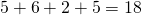Is the sum divisible by 3? Yes. 5,625 is divisble by 3. Is 5,625 divisible by 5 or 10? What is the last digit? It is 5. 5,625 is divisble by 5 but not by 10. Is 5,625 divisible by 6? Is it divisible by both 2 or 3? No, 5,625 is not divisible by 2, so 5,625 is not divisible by 6.

TRY IT 6.1

Determine whether 4,962 is divisible by 2, by 3, by 5, by 6, and by 10

by 2, 3, and 6

TRY IT 6.2

Determine whether 3,765 is divisible by 2, by 3, by 5, by 6, and by 10

by 3 and 5

# Find Prime Factorization and Least Common Multiples

In mathematics, there are often several ways to talk about the same ideas. So far, we’ve seen that if m is a multiple of n, we can say that m is divisible by n. For example, since 72 is a multiple of 8, we say 72 is divisible by 8. Since 72 is a multiple of 9, we say 72 is divisible by 9. We can express this still another way.

Since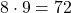, we say that 8 and 9 are factors of 72. When we write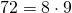, we say we have factored 72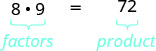Other ways to factor 72 are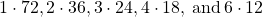. Seventy-two has many factors: 1, 2, 3, 4, 6, 8, 9, 12, 18, 36, and 72

Factors

If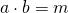, then a and b are factors of m.

Some numbers, like 72, have many factors. Other numbers have only two factors.

Prime Number and Composite Number

A prime number is a counting number greater than 1, whose only factors are 1 and itself.

A composite number is a counting number that is not prime. A composite number has factors other than 1 and itself.

The counting numbers from 2 to 19 are listed in Figure 4, with their factors. Make sure to agree with the “prime” or “composite” label for each!

The prime numbers less than 20 are 2, 3, 5, 7, 11, 13, 17, and 19. Notice that the only even prime number is 2

A composite number can be written as a unique product of primes. This is called the prime factorization of the number. Finding the prime factorization of a composite number will be useful later in this course.

Prime Factorization

The prime factorization of a number is the product of prime numbers that equals the number.

To find the prime factorization of a composite number, find any two factors of the number and use them to create two branches. If a factor is prime, that branch is complete. Circle that prime!

If the factor is not prime, find two factors of the number and continue the process. Once all the branches have circled primes at the end, the factorization is complete. The composite number can now be written as a product of prime numbers.

EXAMPLE 7

Factor 48.
Solution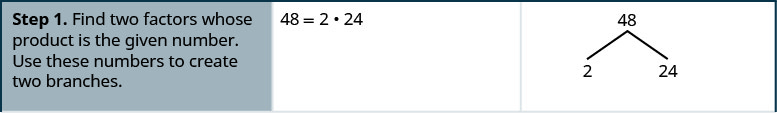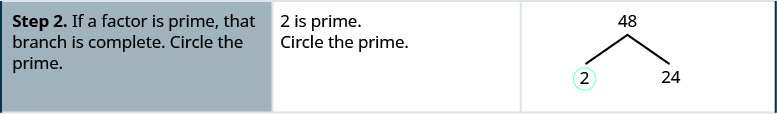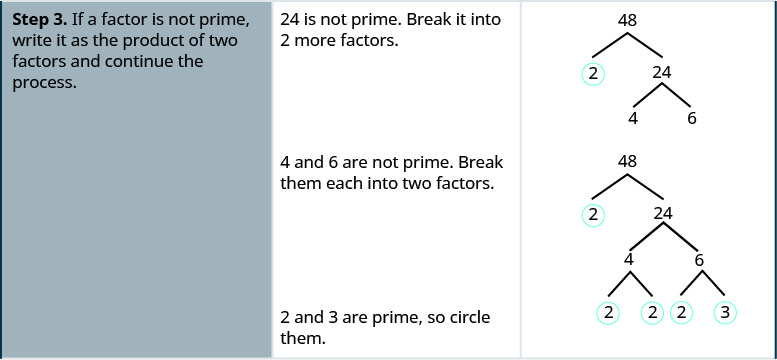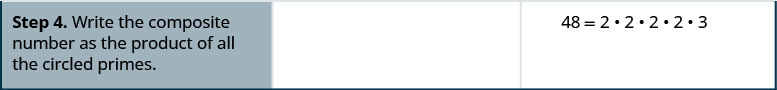We say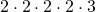is the prime factorization of 48. We generally write the primes in ascending order. Be sure to multiply the factors to verify your answer!

If we first factored 48 in a different way, for example as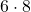, the result would still be the same. Finish the prime factorization and verify this for yourself.

TRY IT 7.1

Find the prime factorization of 80.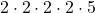TRY IT 7.2

Find the prime factorization of 60.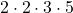HOW TO: Find the Prime Factorization of a Composite Number.

1. Find two factors whose product is the given number, and use these numbers to create two branches.
2. If a factor is prime, that branch is complete. Circle the prime, like a bud on the tree.
3. If a factor is not prime, write it as the product of two factors and continue the process.
4. Write the composite number as the product of all the circled primes.

EXAMPLE 8

Find the prime factorization of 252

Solution
 Step 1. Find two factors whose product is 252. 12 and 21 are not prime. Break 12 and 21 into two more factors. Continue until all primes are factored.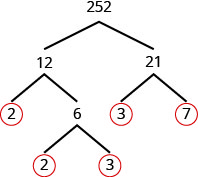Step 2. Write 252 as the product of all the circled primes.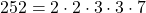TRY IT 8.1

Find the prime factorization of 126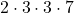TRY IT 8.2

Find the prime factorization of 294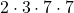One of the reasons we look at multiples and primes is to use these techniques to find the least common multiple of two numbers. This will be useful when we add and subtract fractions with different denominators. Two methods are used most often to find the least common multiple and we will look at both of them.

The first method is the Listing Multiples Method. To find the least common multiple of 12 and 18, we list the first few multiples of 12 and 18: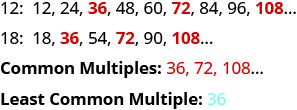Notice that some numbers appear in both lists. They are the common multiples of 12 and 18

We see that the first few common multiples of 12 and 18 are 36, 72, and 108. Since 36 is the smallest of the common multiples, we call it the least common multiple. We often use the abbreviation LCM.

Least Common Multiple

The least common multiple (LCM) of two numbers is the smallest number that is a multiple of both numbers.

The procedure box lists the steps to take to find the LCM using the prime factors method we used above for 12 and 18

HOW TO: Find the Least Common Multiple by Listing Multiples.

1. List several multiples of each number.
2. Look for the smallest number that appears on both lists.
3. This number is the LCM.

EXAMPLE 9

Find the least common multiple of 15 and 20 by listing multiples.

Solution
 Make lists of the first few multiples of 15 and of 20, and use them to find the least common multiple.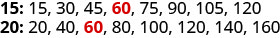Look for the smallest number that appears in both lists. The first number to appear on both lists is 60, so 60 is the least common multiple of 15 and 20.

Notice that 120 is in both lists, too. It is a common multiple, but it is not the least common multiple.

TRY IT 9.1

Find the least common multiple by listing multiples: 9 and 12

36

TRY IT 9.2

Find the least common multiple by listing multiples: 18 and 24

72

Our second method to find the least common multiple of two numbers is to use The Prime Factors Method. Let’s find the LCM of 12 and 18 again, this time using their prime factors.

EXAMPLE 10

Find the Least Common Multiple (LCM) of 12 and 18 using the prime factors method.
Solution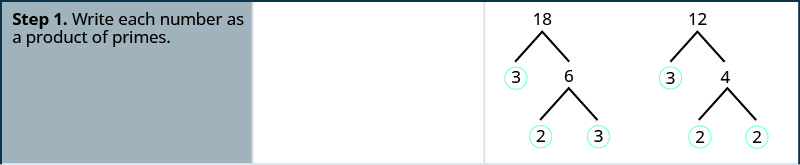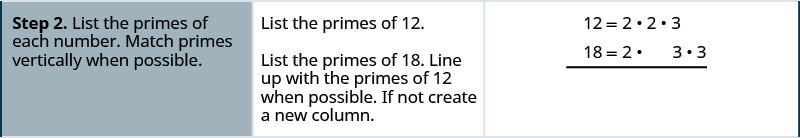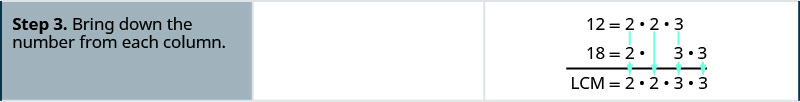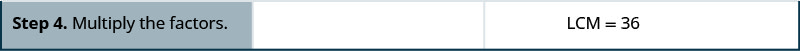Notice that the prime factors of 12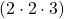and the prime factors of 18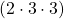are included in the LCM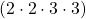. So 36 is the least common multiple of 12 and 18

By matching up the common primes, each common prime factor is used only once. This way you are sure that 36 is the least common multiple.

TRY IT 10.1

Find the LCM using the prime factors method: 9 and 12

36

TRY IT 10.2

Find the LCM using the prime factors method: 18 and 24

72

HOW TO: Find the Least Common Multiple Using the Prime Factors Method.

1. Write each number as a product of primes.
2. List the primes of each number. Match primes vertically when possible.
3. Bring down the columns.
4. Multiply the factors.

EXAMPLE 11

Find the Least Common Multiple (LCM) of 24 and 36 using the prime factors method.

Solution
 Find the primes of 24 and 36. Match primes vertically when possible.Bring down all columns.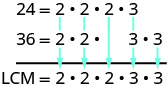Multiply the factors.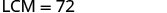The LCM of 24 and 36 is 72.

TRY IT 11.1

Find the LCM using the prime factors method: 21 and 28

84

TRY IT 11.2

Find the LCM using the prime factors method: 24 and 32

96

# Key Concepts

• Place Value as in Figure 2.
• Name a Whole Number in Words
1. Start at the left and name the number in each period, followed by the period name.
2. Put commas in the number to separate the periods.
3. Do not name the ones period.
• Write a Whole Number Using Digits
1. Identify the words that indicate periods. (Remember the ones period is never named.)
2. Draw 3 blanks to indicate the number of places needed in each period. Separate the periods by commas.
3. Name the number in each period and place the digits in the correct place value position.
• Round Whole Numbers
1. Locate the given place value and mark it with an arrow. All digits to the left of the arrow do not change.
2. Underline the digit to the right of the given place value.
3. Is this digit greater than or equal to 5?
• Yes—add 1 to the digit in the given place value.
• No—do not change the digit in the given place value.
4. Replace all digits to the right of the given place value with zeros.
• Divisibility Tests: A number is divisible by:
• 2 if the last digit is 0, 2, 4, 6, or 8.
• 3 if the sum of the digits is divisible by 3.
• 5 if the last digit is 5 or 0.
• 6 if it is divisible by both 2 and 3.
• 10 if it ends with 0.
• Find the Prime Factorization of a Composite Number
1. Find two factors whose product is the given number, and use these numbers to create two branches.
2. If a factor is prime, that branch is complete. Circle the prime, like a bud on the tree.
3. If a factor is not prime, write it as the product of two factors and continue the process.
4. Write the composite number as the product of all the circled primes.
• Find the Least Common Multiple by Listing Multiples
1. List several multiples of each number.
2. Look for the smallest number that appears on both lists.
3. This number is the LCM.
• Find the Least Common Multiple Using the Prime Factors Method
1. Write each number as a product of primes.
2. List the primes of each number. Match primes vertically when possible.
3. Bring down the columns.
4. Multiply the factors.

# Glossary

composite number
A composite number is a counting number that is not prime. A composite number has factors other than 1 and itself.
counting numbers
The counting numbers are the numbers 1, 2, 3, …
divisible by a number
If a number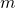is a multiple of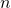, thenis divisible by. (If 6 is a multiple of 3, then 6 is divisible by 3.)
factors
If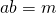, then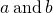are factors of. Since 3 · 4 = 12, then 3 and 4 are factors of 12.
least common multiple
The least common multiple of two numbers is the smallest number that is a multiple of both numbers.
multiple of a number
A number is a multiple of n if it is the product of a counting number and n.
number line
A number line is used to visualize numbers. The numbers on the number line get larger as they go from left to right, and smaller as they go from right to left.
origin
The origin is the point labeled 0 on a number line.
prime factorization
The prime factorization of a number is the product of prime numbers that equals the number.
prime number
A prime number is a counting number greater than 1, whose only factors are 1 and itself.
whole numbers
The whole numbers are the numbers 0, 1, 2, 3, ….

# Practice Makes Perfect

## Use Place Value with Whole Numbers

In the following exercises, find the place value of each digit in the given numbers.

 1. 51,493 a) 1 b) 4 c) 9 d) 5 e) 3 2. 87,210 a) 2 b) 8 c) 0 d) 7 e) 1 3. 164,285 a) 5 b) 6 c) 1 d) 8 e) 2 4. 395,076 a) 5 b) 3 c) 7 d) 0 e) 9 5. 93,285,170 a) 9 b) 8 c) 7 d) 5 e) 3 6. 36,084,215 a) 8 b) 6 c) 5 d) 4 e) 3 7. 7,284,915,860,132 a) 7 b) 4 c) 5 d) 3 e) 0 8. 2,850,361,159,433 a) 9 b) 8 c) 6 d) 4 e) 2

In the following exercises, name each number using words.

 9. 1,078 10. 5,902 11. 364,510 12. 146,023 13. 5,846,103 14. 1,458,398 15. 37,889,005 16. 62,008,465

In the following exercises, write each number as a whole number using digits.

 17. four hundred twelve 18. two hundred fifty-three 19. thirty-five thousand, nine hundred seventy-five 20. sixty-one thousand, four hundred fifteen 21. eleven million, forty-four thousand, one hundred sixty-seven 22. eighteen million, one hundred two thousand, seven hundred eighty-three 23. three billion, two hundred twenty-six million, five hundred twelve thousand, seventeen 24. eleven billion, four hundred seventy-one million, thirty-six thousand, one hundred six

In the following, round to the indicated place value.

 25. Round to the nearest ten. a) 386 b) 2,931 26. Round to the nearest ten. a) 792 b) 5,647 27. Round to the nearest hundred. a) 13,748 b) 391,794 28. Round to the nearest hundred. a) 28,166 b) 481,628 29. Round to the nearest ten. a) 1,492 b) 1,497 30. Round to the nearest ten. a) 2,791 b) 2,795 31. Round to the nearest hundred. a) 63,994 b) 63,940 32. Round to the nearest hundred. a) 49,584 b) 49,548

In the following exercises, round each number to the nearest a) hundred, b) thousand, c) ten thousand.

 33. 392,546 34. 619,348 35. 2,586,991 36. 4,287,965

## Identify Multiples and Factors

In the following exercises, use the divisibility tests to determine whether each number is divisible by 2, 3, 5, 6, and 10

 37. 84 38. 9,696 39. 75 40. 78 41. 900 42. 800 43. 986 44. 942 45. 350 46. 550 47. 22,335 48. 39,075

## Find Prime Factorizations and Least Common Multiples

In the following exercises, find the prime factorization.

 49. 86 50. 78 51. 132 52. 455 53. 693 54. 400 55. 432 56. 627 57. 2,160 58. 2,520

In the following exercises, find the least common multiple of the each pair of numbers using the multiples method.

 59. 8, 12 60. 4, 3 61. 12, 16 62. 30, 40 63. 20, 30 64. 44, 55

In the following exercises, find the least common multiple of each pair of numbers using the prime factors method.

 65. 8, 12 66. 12, 16 67. 28, 40 68. 84, 90 69. 55, 88 70. 60, 72

## Everyday Math

 71. Writing a Check Jorge bought a car for $24,493. He paid for the car with a check. Write the purchase price in words. 72. Writing a Check Marissa’s kitchen remodeling cost$18,549. She wrote a check to the contractor. Write the amount paid in words. 73. Buying a Car Jorge bought a car for $24,493. Round the price to the nearest a) ten b) hundred c) thousand; and d) ten-thousand. 74. Remodeling a Kitchen Marissa’s kitchen remodeling cost$18,549, Round the cost to the nearest a) ten b) hundred c) thousand and d) ten-thousand. 75. Population The population of China was 1,339,724,852 on November 1, 2010. Round the population to the nearest a) billion b) hundred-million; and c) million. 76. Astronomy The average distance between Earth and the sun is 149,597,888 kilometres. Round the distance to the nearest a) hundred-million b) ten-million; and c) million. 77. Grocery Shopping Hot dogs are sold in packages of 10, but hot dog buns come in packs of eight. What is the smallest number that makes the hot dogs and buns come out even? 78. Grocery Shopping Paper plates are sold in packages of 12 and party cups come in packs of eight. What is the smallest number that makes the plates and cups come out even?

## Writing Exercises

 79. What is the difference between prime numbers and composite numbers? 80. Give an everyday example where it helps to round numbers. 81. Explain in your own words how to find the prime factorization of a composite number, using any method you prefer.

 1. a) thousands b) hundreds c) tens d) ten thousands e) ones 3. a) ones b) ten thousands c) hundred thousands d) tens e) hundreds 5. a) ten millions b) ten thousands c) tens d) thousands e) millions 7. a) trillions b) billions c) millions d) tens e) thousands 9. one thousand, seventy-eight 11. three hundred sixty-four thousand, five hundred ten 13. five million, eight hundred forty-six thousand, one hundred three 15. thirty-seven million, eight hundred eighty-nine thousand, five 17. 412 19. 35,975 21. 11,044,167 23. 3,226,512,017 25. a) 390 b) 2,930 27. a) 13,700 b) 391,800 29. a) 1,490 b) 1,500 31. a) 64,000 b) 63,900 33. a) 392,500 b) 393,000 c) 390,000 35. a) 2,587,000 b) 2,587,000 c) 2,590,000 37. divisible by 2, 3, and 6 39. divisible by 3 and 5 41. divisible by 2, 3, 5, 6, and 10 43. divisible by 2 45. divisible by 2, 5, and 10 47. divisible by 3 and 5 49.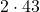51.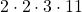53.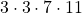55.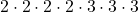57.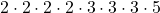59. 24 61. 48 63. 60 65. 24 67. 420 69. 440 71. twenty-four thousand, four hundred ninety-three dollars 73. a) $24,490 b)$24,500 c) $24,000 d)$20,000 75. a) 1,000,000,000 b) 1,300,000,000 c) 1,340,000,000 77. 40 79. Answers may vary. 81. Answers may vary.